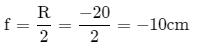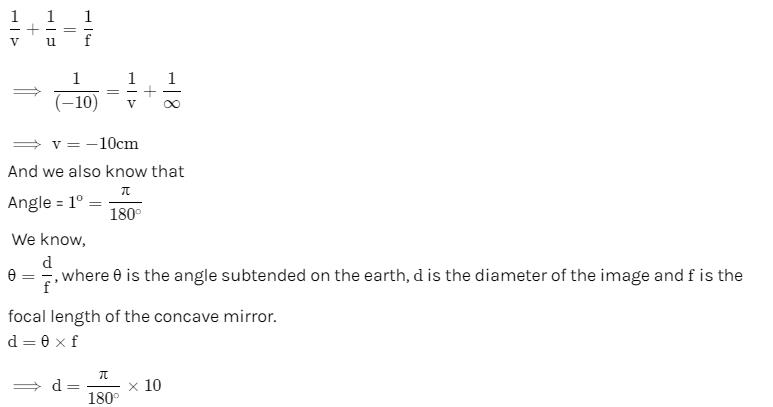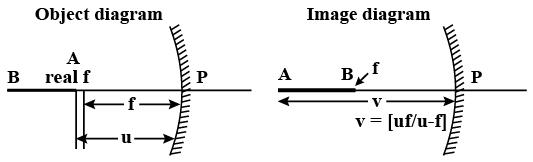Courses

# Test: Geometric Optics (Competition Level 1),

## 30 Questions MCQ Test Physics Class 12 | Test: Geometric Optics (Competition Level 1),

Description
This mock test of Test: Geometric Optics (Competition Level 1), for Class 12 helps you for every Class 12 entrance exam. This contains 30 Multiple Choice Questions for Class 12 Test: Geometric Optics (Competition Level 1), (mcq) to study with solutions a complete question bank. The solved questions answers in this Test: Geometric Optics (Competition Level 1), quiz give you a good mix of easy questions and tough questions. Class 12 students definitely take this Test: Geometric Optics (Competition Level 1), exercise for a better result in the exam. You can find other Test: Geometric Optics (Competition Level 1), extra questions, long questions & short questions for Class 12 on EduRev as well by searching above.
QUESTION: 1

Solution:
QUESTION: 2

Solution:
QUESTION: 3

### A watch shows the time as 3 : 25. What will be the time that appears when seen through a plane mirror ?

Solution:
QUESTION: 4

If a ray of light is incident on a plane mirror at an angle 60° from the mirror surface, then deviation produced by mirror is

Solution:

According to the law of reflection, the angle of incidence and angle of reflection are equal. Both these angles are measured with respect to a line normal to the reflection surface. If I understand the question correctly, the incident ray makes an angle of 60 degrees with the mirror surface, then the angle of incidence can be calculated as:

Angle of incidence = 90 - angle made with the mirror surface

= 90 - 60 = 30 degrees.

Since, angle of incidence = angle of reflection = 30 degrees.

The incident ray will have an angle of reflection of 30 degrees (made with a surface normal to the mirror surface).

The reflected ray will make an angle of 60 degrees (90 - 30 degrees) with the mirror surface.

QUESTION: 5

When light is reflected from a mirror a change occurs in its

Solution:
QUESTION: 6

The images of clouds and trees in water always less bright than in reality

Solution:
QUESTION: 7

A rays is incident at an angle 38º on a mirror. The angle between normal and reflected ray is

Solution:
QUESTION: 8

Mark the correct options

Solution:
QUESTION: 9

A point source of light is placed in front of a plane mirror.

Solution:
QUESTION: 10

Which of the following letters do not surface lateral inversion

Solution:
QUESTION: 11

An object is initially at a distance of 100 cm from a plane mirror. If the mirror approaches the object at a speed of 5 cm/s. Then after 6 s the distance between the object and its image will be

Solution:
QUESTION: 12

Two mirrors are placed perpendicular to each other. A ray strikes the first mirror and after reflection from the first mirror it falls on the second mirror. The ray after reflection from second mirror will emerge

Solution:
QUESTION: 13

A person is in a room whose ceiling and two adjacent walls are mirrors. How many images are formed?

Solution:

The number of images formed when two mirrors are inclined to each other is given by :
n=(360/θ -1)
here θ= 90°[since walls are perpendicular]
so, number of images=n=360/90-1
=4-1
=3
These 3 images are formed by a combination of two adjacent walls with the object itself acts as objects for the ceiling mirror. So totally 4 images are formed.
Hence total number of images formed are 4+3= 7

QUESTION: 14

If an object is placed unsymmetrically between two plane mirrors, inclined at the angle of 600, then the total number of images formed is

Solution:
QUESTION: 15

In image formation from spherical mirrors, only paraxial rays are considered because they

Solution:
QUESTION: 16

A concave mirror of radius of curvature 20 cm forms image of the sun. The diameter of the sun subtends an angle 1° on the earth. Then the diameter of the image is (in cm)

Solution:

Given: A concave mirror of radius of curvature 20cm forms image of the sun. The diameter of the sun subtends an angle 1o on the earth.

To find the diameter of the image

Solution:

As sun is more far from the earth,

Then we take u = infinite

Focal length,From the mirror formulad = π/18

QUESTION: 17

A convex mirror has a focal length f. A real object, placed at a distance f in front of it from the pole, produces an image at

Solution:
QUESTION: 18

A convex mirror has a focal length = 20 cm. A convergent beam tending to converge to a point 20 cm behind convex mirror on principal axis falls on it. The image if formed at

Solution:
QUESTION: 19

An object is placed at a distance u from a concave mirror and its real image is received on a screen placed at a distance of v from the mirror. If f is the focal length of the mirror, then the graph between 1/v versus 1/u is

Solution:
QUESTION: 20

An infinitely long rod lies along the axis of a concave mirror of focal length f. The near end of the rod is at a distance u > f from the mirror. Its image will have a length

Solution:

At infinity, image of B will form at focus since it is at infinity. Image of A will be at A which can be calculated by mirror formula.
1/v​+(1/−u)​=(1/−f)
⟹v= fu /(f−u)​=−( fu/(u−f)​)
Image length = v−f=(fu/(u−f)​)−(f)= f2​/(u−f)
(We take the absolute values of the distances to calculate the rod length)QUESTION: 21

The radius of curvature for a convex lens is 40 cm, for each surface. Its refractive index is 1.5. The focal length will be

Solution:
QUESTION: 22

A concave mirror gives an image three times as large as the object placed at a distance of 20 cm from it. For the image to be real, the focal length should be

Solution:
QUESTION: 23

If an object is 30 cm away from a concave mirror of focal length 15 cm, the image will be

Solution:
QUESTION: 24

A concave mirror cannot form

Solution:
QUESTION: 25

The largest distance of the image of a real object from a convex mirror of focal length 20 cm can be

Solution:
QUESTION: 26

A particle is moving towards a fixed spherical mirror. The image :

Solution:

1/v + 1/u = 1/f
⇒(-1/v2)x(dv/dt)-(1/u2)(du/dt)=0
dv/dt=-(v2/u2)(du/dt)
For spherical mirror: u is negative and particle is moving towards mirror i.e. u is increasing and du/dt is positive, therefore dv/dt must be negative or v should decrease.
Case-1 ⇒ Image real ⇒ v negative ⇒ decreasing v means image moving away from mirror
Case-2 ⇒ Image virtual ⇒ v positive ⇒ decreasing v means image moving towards mirror
So, image may move towards the mirror

QUESTION: 27

A straight line joining the object point and image point is always perpendicular to the mirror

Solution:
QUESTION: 28

The focal length of spherical mirror is

Solution:
QUESTION: 29

A virtual image, larger than the object can be produced by

Solution:
QUESTION: 30

In case of concave mirror, the minimum distance between a real object and its real image is :

Solution: